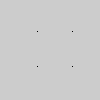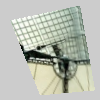Name

### vertex()

Examples```beginShape(POINTS)
vertex(30, 20)
vertex(85, 20)
vertex(85, 75)
vertex(30, 75)
endShape()
``````# Drawing vertices in 3D requires P3D
# as a parameter to size()
size(100, 100, P3D)
beginShape(POINTS)
vertex(30, 20, -50)
vertex(85, 20, -50)
vertex(85, 75, -50)
vertex(30, 75, -50)
endShape()
``````size(100, 100, P3D)
noStroke()
beginShape()
texture(img)
# "laDefense.jpg" is 100x100 pixels in size so
# the values 0 and 100 are used for the
# parameters "u" and "v" to map it directly
# to the vertex points
vertex(10, 20, 0, 0)
vertex(80, 5, 100, 0)
vertex(95, 90, 100, 100)
vertex(40, 95, 0, 100)
endShape()
```
Description All shapes are constructed by connecting a series of vertices. vertex() is used to specify the vertex coordinates for points, lines, triangles, quads, and polygons. It is used exclusively within the beginShape() and endShape() functions.

Drawing a vertex in 3D using the z parameter requires the P3D parameter in combination with size, as shown in the above example.

This function is also used to map a texture onto geometry. The texture() function declares the texture to apply to the geometry and the u and v coordinates set define the mapping of this texture to the form. By default, the coordinates used for u and v are specified in relation to the image's size in pixels, but this relation can be changed with textureMode().
Related beginShape()
endShape()
bezierVertex()